### Dijkstra's Algorithm

A common example of a graph-based pathfinding algorithm is Dijkstra's algorithm. This algorithm begins with a start node and an "open set" of candidate nodes. At each step, the node in the open set with the lowest distance from the start is examined. The node is marked "closed", and all nodes adjacent to it are added to the open set if they have not already been examined. This process repeats until a path to the destination has been found. Since the lowest distance nodes are examined first, the first time the destination is found, the path to it will be the shortest path.

Dijkstra's algorithm fails if there is a negative edge weight. In the hypothetical situation where Nodes A, B, and C form a connected undirected graph with edges AB = 3, AC = 4, and BC = -2, the optimal path from A to C costs 1, and the optimal path from A to B costs 2. Dijkstra's Algorithm starting from A will first examine B, as that is the closest. It will assign a cost of 3 to it, and mark it closed, meaning that its cost will never be reevaluated. Therefore, Dijkstra's cannot evaluate negative edge weights. However, since for many practical purposes there will never be a negative edgeweight, Dijkstra's algorithm is largely suitable for the purpose of pathfinding.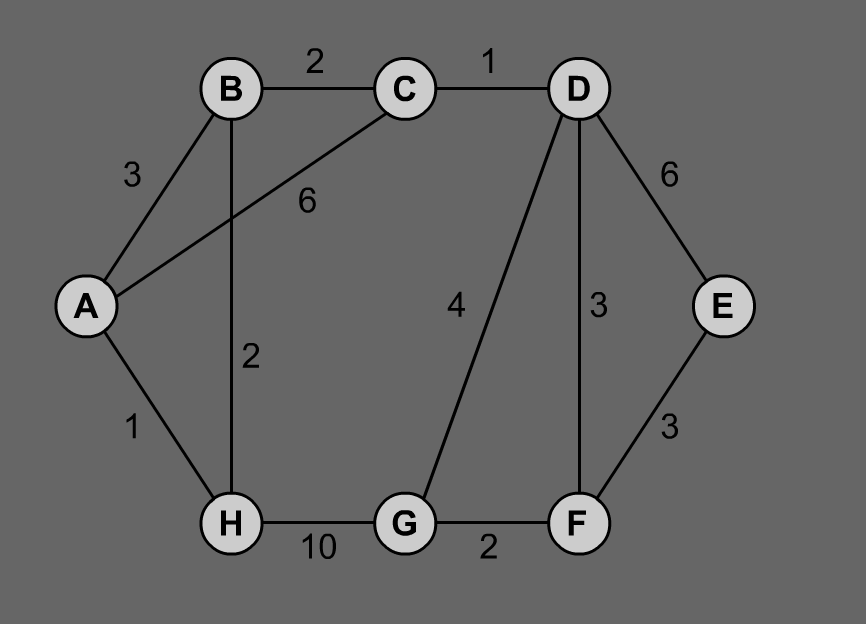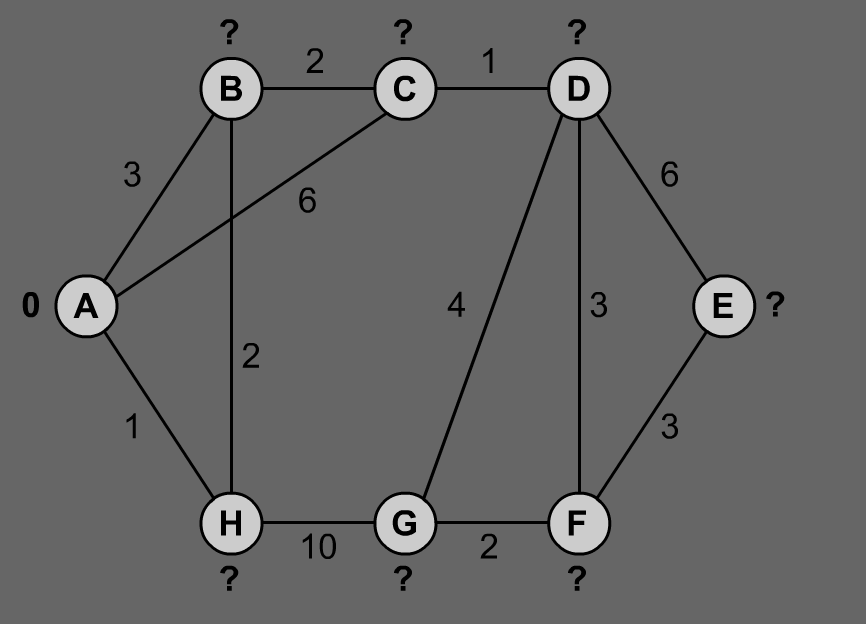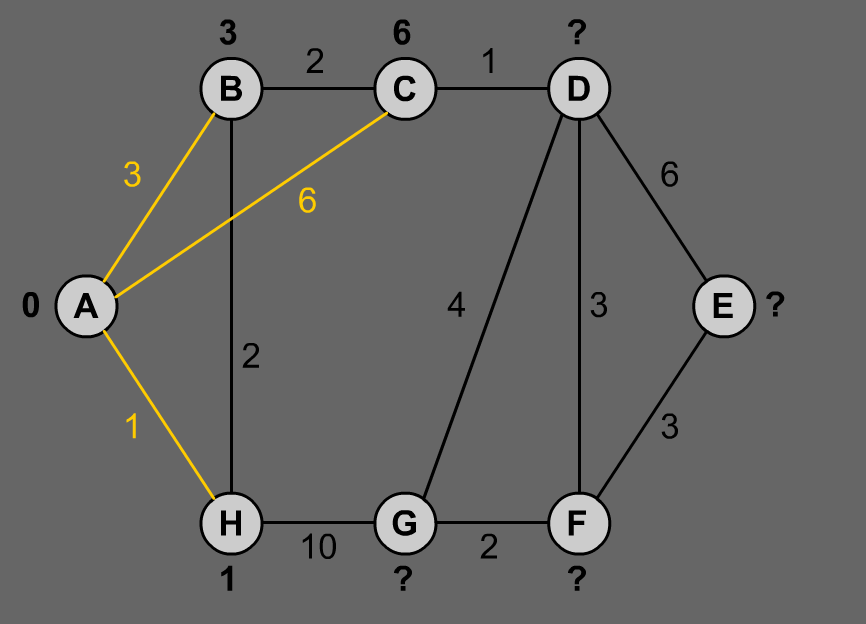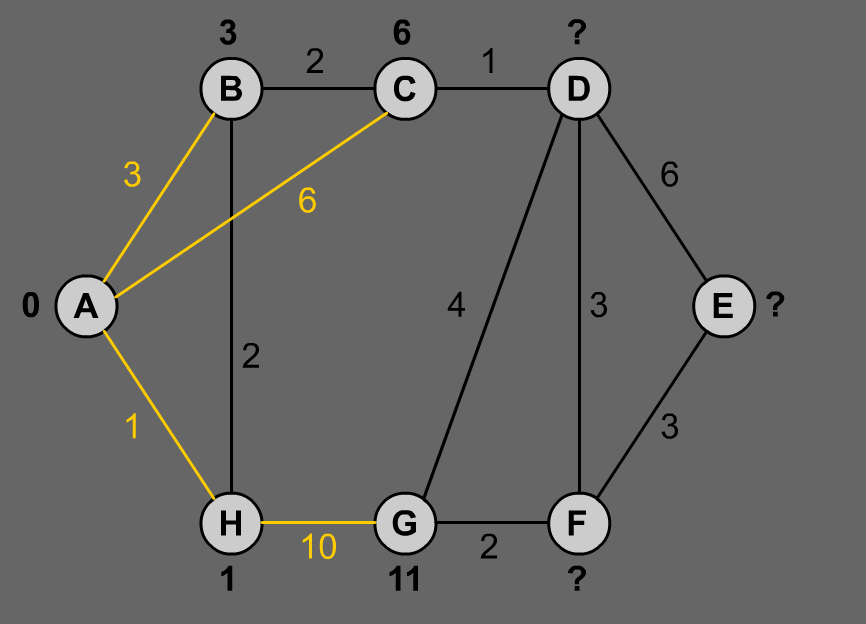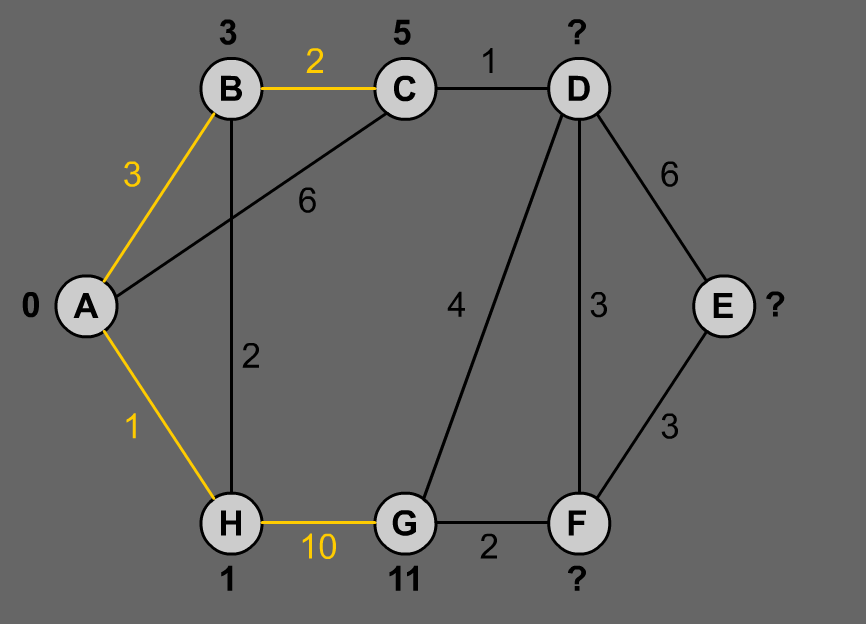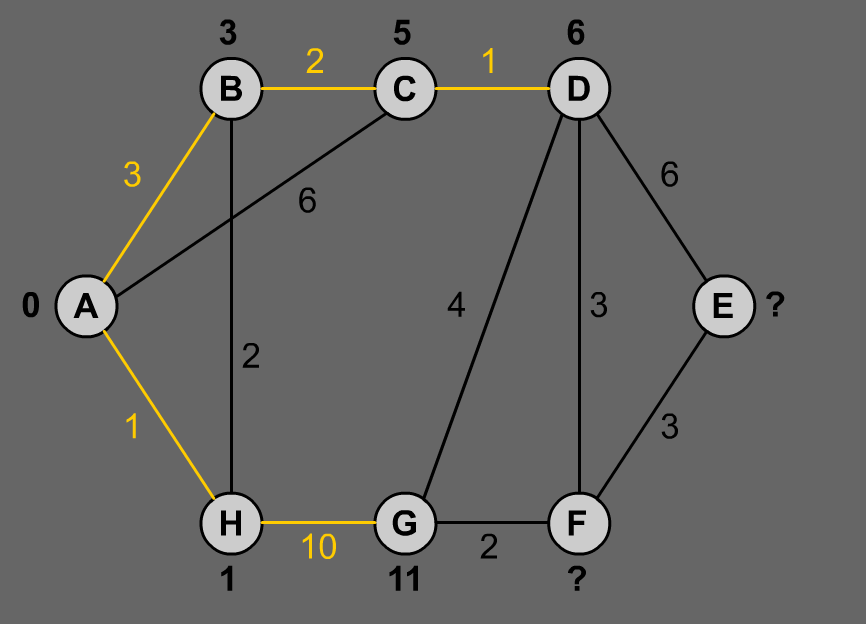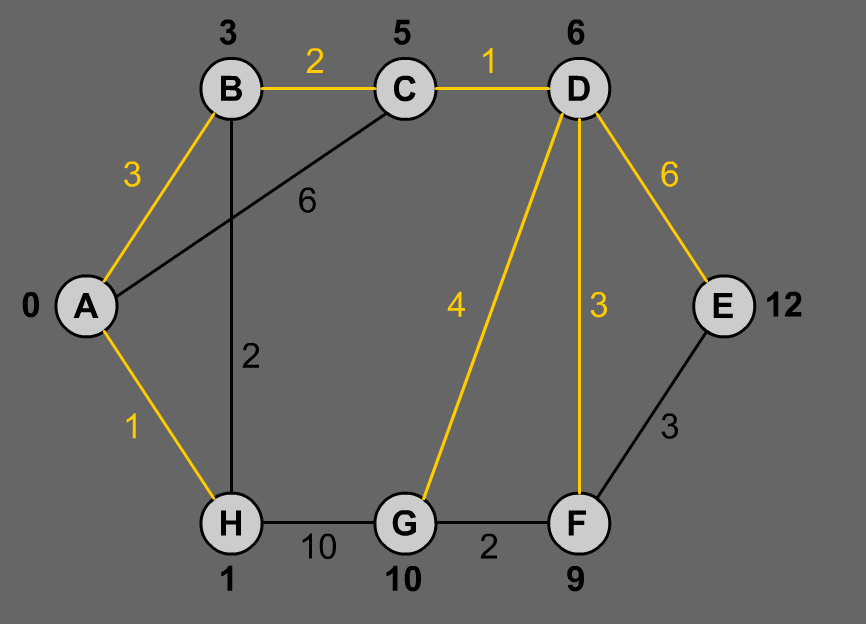Dijkstra's algorithm runtime. It picks the unvisited vertex with the lowest distance, calculates the distance through it to each unvisited neighbor, and updates the neighbor's distance if smaller.

 ```procedure DIJKSTRA(G, s)     begin         for each node v from G.V do             begin                 d[v] := ?                 ancestor[v] := NIL             end         d[s] := 0         INSERT_NODES(Q, G.V)         while not (EMPTY(Q)) do             begin                 u := MIN(Q)                 for each node v from G.Neighbours(u) do                     if d[v] > d[u] + G.P[(u, v)].weight then                         begin                             d[v] := d[u] + G.P[(u, v)].weight                             ancestor[v] := u                         end             end     end procedure PATH(G, s, v)     begin         if v = s then             print s + ": lenght: " + G.d[s]         else if G.ancestor[v] = NIL then             print "between " + s + " and " + v + " is no path"         else             begin                 PATH(G, s, G.ancestor[v])                 print v + ": lenght: " + G.d[v]             end     end```

### A* Algorithm

Pathfinding algorithm A* was developed by Nils Nilsson, Bertram Raphael and Peter E. Hart in 1968 as an upgrade of Dijkstra's algorithm so a computer program could find only one shortest path over obstacles to the chosen point instead of searching multiple shortest paths to all possible points in the area like Dijkstra's algorithm did. It has proven to be faster and soon it found its way in artificial intelligence in computer games. But because virtual environments are different from game to game, careless implementation regarding the pseudocode cannot be sufficient. It is necessary to understand when and in what situation procedures and functions must run to perform the shortest path search. Computer game developers face this problem constantly and so we can witness products with bad and good artificial intelligence.

A* is a variant of Dijkstra's algorithm commonly used in games. A* assigns a weight to each open node equal to the weight of the edge to that node plus the approximate distance between that node and the finish. This approximate distance is found by the heuristic, and represents a minimum possible distance between that node and the end. This allows it to eliminate longer paths once an initial path is found. If there is a path of length x between the start and finish, and the minimum distance between a node and the finish is greater than x, that node need not be examined.

A* uses this heuristic to improve on the behavior relative to Dijkstra's algorithm. When the heuristic evaluates to zero, A* is equivalent to Dijkstra's algorithm. As the heuristic estimate increases and gets closer to the true distance, A* continues to find optimal paths, but runs faster (by virtue of examining fewer nodes). When the value of the heuristic is exactly the true distance, A* examines the fewest nodes. (However, it is generally impractical to write a heuristic function that always computes the true distance.) As the value of the heuristic increases, A* examines fewer nodes but no longer guarantees an optimal path. In many applications (such as video games) this is acceptable and even desirable, in order to keep the algorithm running quickly.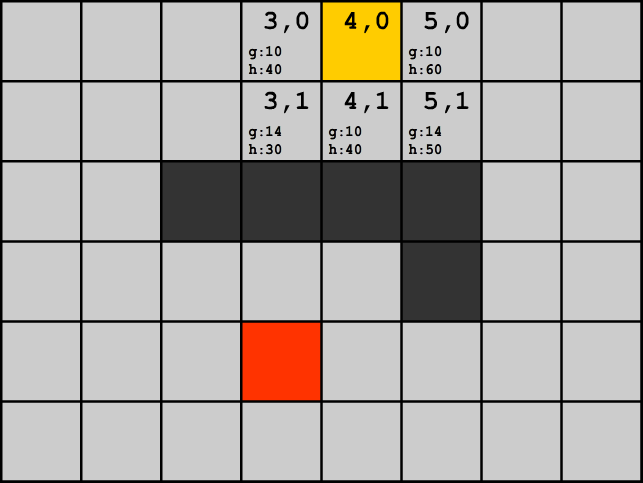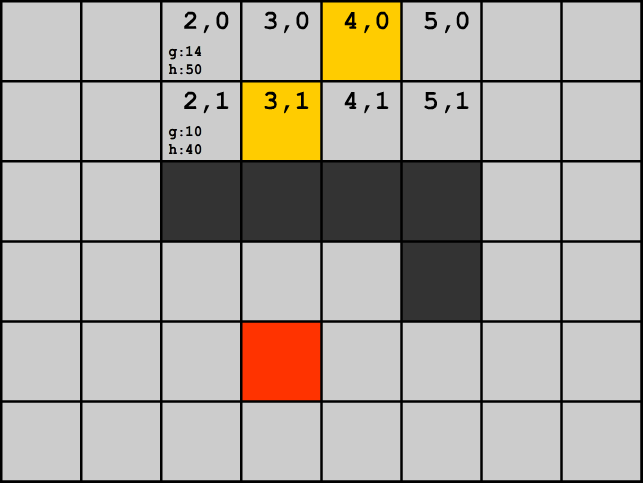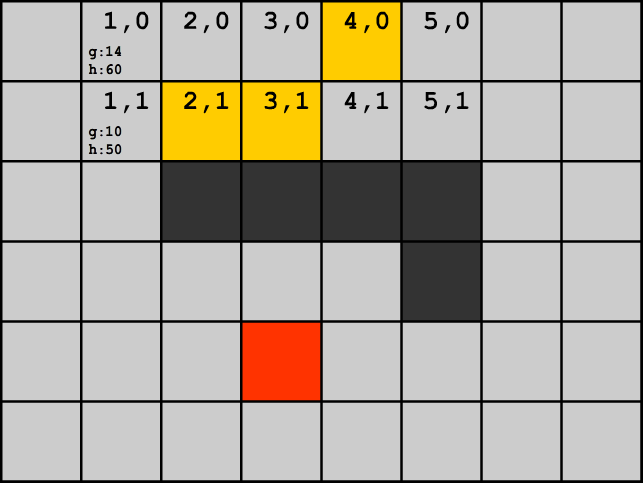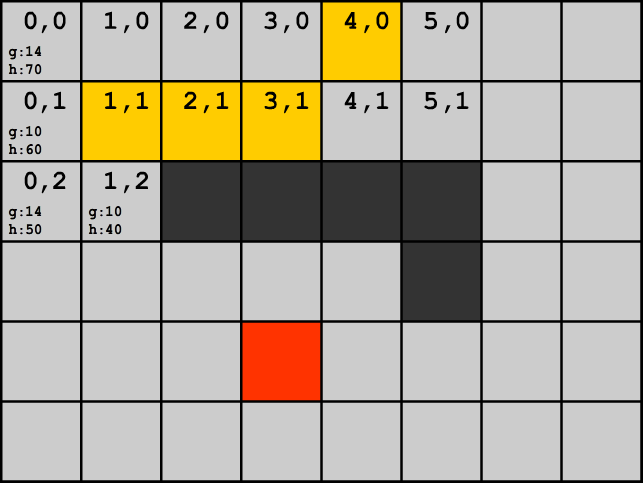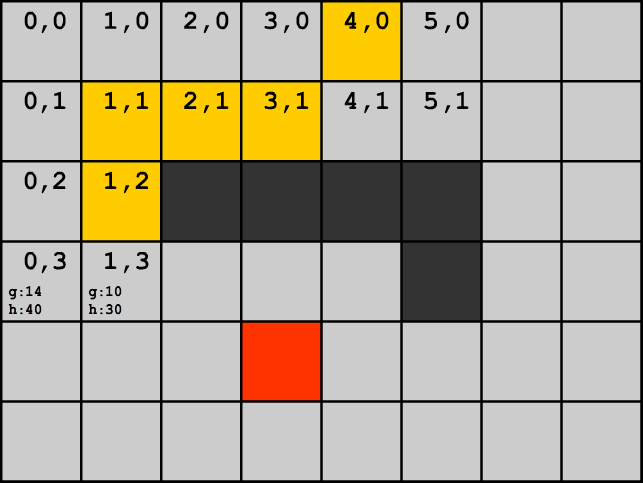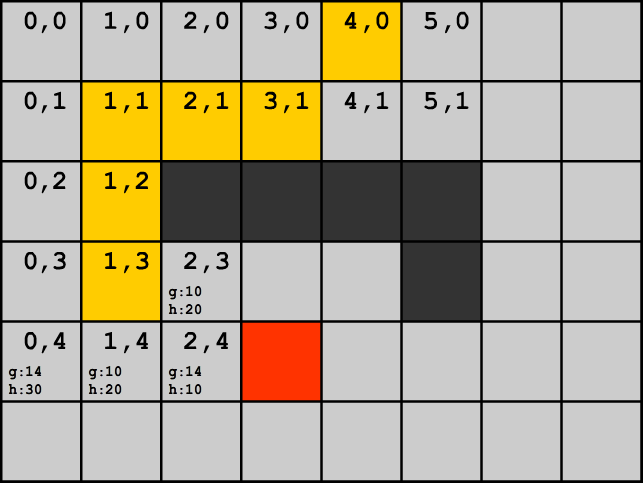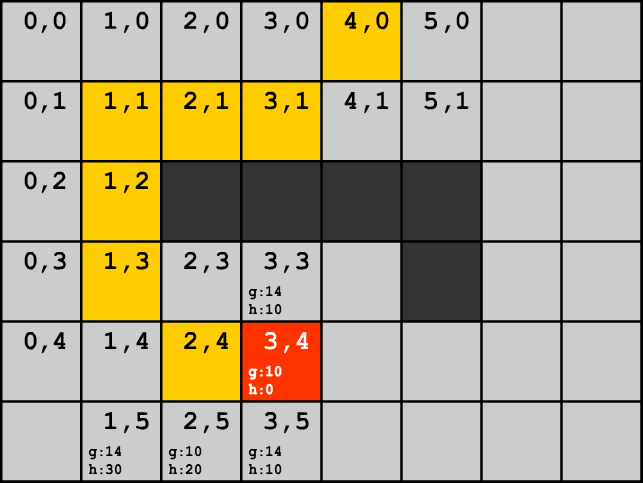A* pathfinding algorithm navigating around the obstacle and searching for the shortest path between the source and destination.

 ```struct node     x     y     g     h     predecessor openNodes[] = 0 closeNodes[] = 0 shortestPath[] = 0 node = (start_X, start_Y, 0, 0, null) openNodes[start_X, start_Y] = node A_star() funkcija A_star()     currentNode     min_evaluation_F = 100000     for each (node in openNodes)         current_evaluation_F = node.g + node.h         if (min_evaluation_F > current_evaluation_F)             min_evaluation_F = current_evaluation_F             currentNode = node     if (currentNode == null)         return     delete openNodes[currentNode.x, currentNode.y]     closeNodes[currentNode.x, currentNode.y] = currentNode     if (currentNode.x == destination_X && currentNode.y == destination_Y)         destinationNode = currentNode         final = true     for (neighbour in neighbourstvo(currentNode))         if (neighbour != obstacle && closeNodes[neighbour.x, neighbour.y] == null && openNodes[neighbour.x, neighbour.y] == null)             g = 10             if (diagonally(neighbour))                 g = 14             h = (|neighbour.x - destination_X| + |neighbour.y - destination_Y|) * 10             new_node = (neighbour.x, neighbour.y, g, h, currentNode)             openNodes[neighbour.x, neighbour.y] = new_node     if (!final)         A_star()     else         printPath(destinationNode) funkcija printPath(node)     move = (node.x, node.y)     shortestPath.push(move)     if (node.g > 0)         printPath(node.predecessor)```

### Direction oriented pathfinding in video games

Authors:

Xiao Cui is a Ph.D student in School of Enignnering and Science at Victoria University, Australia. He completed his master degree in the area of Software Engineering at Australian National University and obtained his Bachelor of Computer Science degree at Victoria University. His research interests include object-oriented software engineering, pathfinding algorithms, database management and game programming.

Hao Shi is an Associate Professor in School of Engineering and Science at Victoria University, Australia. She completed her PhD in the area of Computer Engineering at University of Wollongong and obtained her Bachelor of Engineering degree at Shanghai Jiao Tong University, China. She has been actively engaged in R&D and external consultancy activities. Her research interests include p2p Network, Location-Based Services, Web Services, Computer/Robotics Vision, Visual Communications, Internet and Multimedia Technologies.

Pathfinding generally refers to find an optimal path between any two locations. In the context of video games, an optimal path between any two locations is the least cost path rather than the shortest path. That means a path along the road may be better than a shorter path through a hill in terms of time as the cost of climbing a hill is obviously higher than the cost of walking along a road. However, the actual game world as shown in Figure 1a doesn't contain such information. The general solution is to overlay another map with cost information on the actual game world as shown in Figure 1b and adopt a search algorithm on it to find the least cost path. Current solutions for pathfinding, in the context of video games, either provide a high speed search by sacrificing accuracy or produce an optimal path but using more time and resources. How to find an optimal path more efficiently is still an area of study.

Dijkstra's algorithm and A* algorithm are two classic graph search algorithms in geometry, which can find the shortest path between any two nodes on a weighted graph as shown in Figure 2. In weighted graphs, the shortest path exactly equals the least cost path. That means finding an optimal path on a weighted graph equals finding the shortest path on it.

In the past, Dijkstra's algorithm was the only choice for pathfinding until last two decades A* algorithm had become the mainstream solution for pathfinding in video games. Dijkstra's algorithm can find the shortest path from the source node to all the other nodes on the graph and A* algorithm produces the shortest path for a pair of nodes on the graph.

Unlike Dijkstra's algorithm where an exhaustive search of the entire graph is required as shown in Figure 3a, A* algorithm uses a heuristic method to reduce the research space as shown in Figure 3b. That means only the promising nodes are examined. Figure 4 shows an example of how a heuristic method can affect the search space.

### Path-finding and Terrain Analysis

Author:

Ruben Tous
Universitat Pompeu Fabra (UPF), Departament de Tecnologia,
Pg. Circumvallacio, 8. E-08003 Barcelona, Spain
ruben.tous@tecn.upf.es

Path-finding is, of all the decisions involved in game's AI, probably the most common. The problem consists in looking for a good route when moving an entity from here to there. The entity can be any mobile entity of the game (a virtual character, a vehicle, etc.). A good route means a realistic route (that respects the terrain topology), and a route that minimizes the cost (energy, food, etc.). Here it must be remarked that even we're using the term "path-finding" and "path-planning" in the same way, these two terms mean different things. Path-planning is the action of deciding which route to take, based on and expressed in terms of the current internal representation of the terrain, while pathfinding is the execution of this theoretical route, by translating the plan from the internal representation in terms of physical movement in the environment. Pathfinding can appear in games of any genre (action games, simulators, role-playing, strategy, etc.) where the computer is responsible for moving things around. It could seem a trivial problem, because is as old as games themselves, but questions about path-finding are regularly seen in online game programming forums, and the entities in several nowadays games move in less than intelligent paths.

Recently pathfinding is included in a bigger sack called Terrain Analysis. This term, overall used when referring to RTS games, refers to any kind of strategy that involves units movements, and can go from simple pathfinding in tile maps to more complex issues like area decomposition or influence maps. It reflects the necessity to reduce the gap between the way human player and computers act. Though this issue is very interesting and introduces the concept of abstraction (for example in area decomposition) when facing the pathfinding problem, it's not the target of this article. Here we will focus on remarking that recent research in the field of AI Planning will serve as an alternative way to A* when solving the classic pathfinding problem.

### Path-finding Sample: Real Time Strategy (RTS) Games

Author:

Ruben Tous
Universitat Pompeu Fabra (UPF), Departament de Tecnologia,
Pg. Circumvallacio, 8. E-08003 Barcelona, Spain
ruben.tous@tecn.upf.es

RTS is one of the most popular genres of computer games nowadays. It appeared more than ten years ago with games like Herzog Zwei (1989) or Westwood's Dune II (1992), and consolidated with titles like Blizzard's Warcraft (1994) or Westwood's Command&Conquer (1995). The target of a RTS game is to coordinate a relatively big amount of partially autonomous units (workers, soldiers, vehicles,...) to overcome one or more players, computer or human, with their own groups of units over a shared territory. In a RTS game the units move autonomously, but the player can order certain unit to move to any certain location. Unit movements, autonomous or not, need to be managed by the computer, and here is where comes path-finding. The territory of a RTS game seems continuous, but it isn't, it is divided in cells approximately of the size of an unit. Units can only move according to the cell grid. So this is a classical path-finding problem but with two added complexities: 1) Uncertainty: Other moving entities could block the way and invalidate the previously computed path-plan. 2) Limited CPU: Hundreds or even thousands of units are moving at the same time and need to compute their next move.